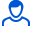Content: K3-07.pdf (219.88 KB)

Positive responses: -1
Negative responses: 0

Sold: -1
Refunds: -1Loyalty discount! If the total amount of your purchases from the seller more than:

 \$1 the discount is 1% \$5 the discount is 2% \$10 the discount is 3% \$20 the discount is 5% \$50 the discount is 7% \$100 the discount is 10% \$200 the discount is 15%

K3-07 Dievskiy

For the mechanism shown in the diagram, consisting of a connecting rod AB of 2 m in length and two sliders, the speed and acceleration of the slider A determine the speed and acceleration of the slider B and the middle point C of the connecting rod, as well as the angular velocity and the angular acceleration of the connecting rod.
Formatted in .pdf
No feedback yet